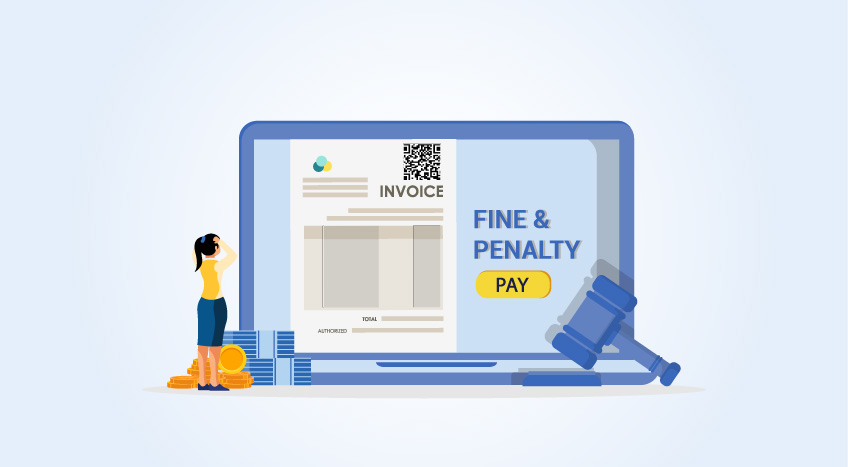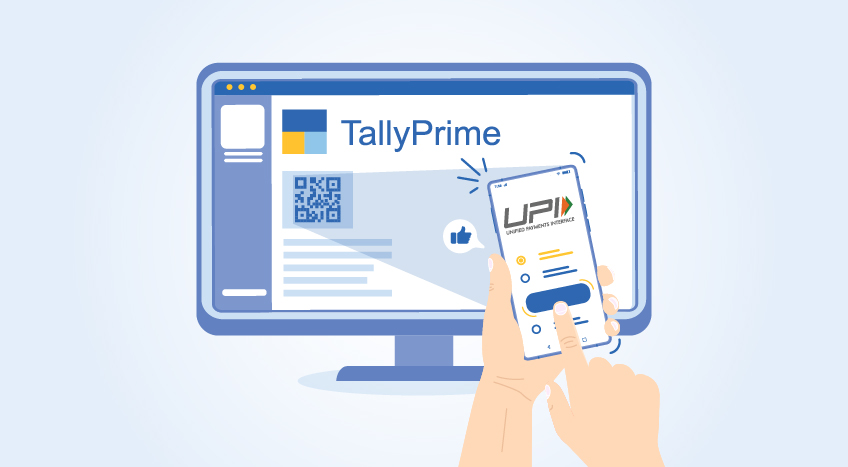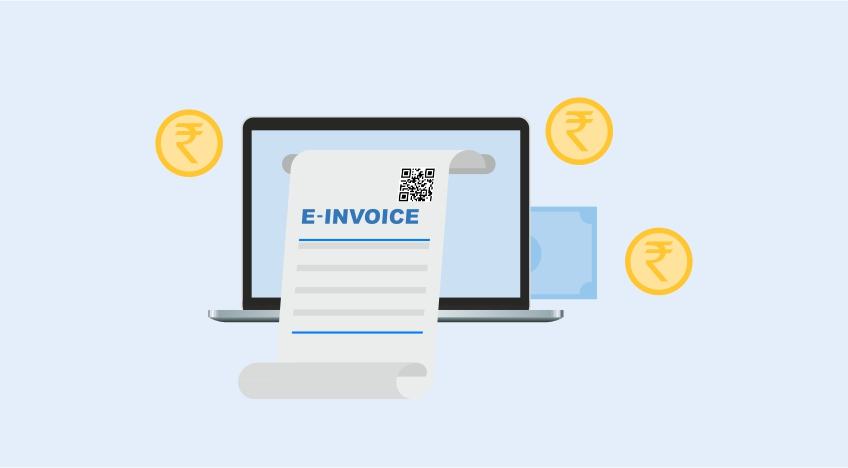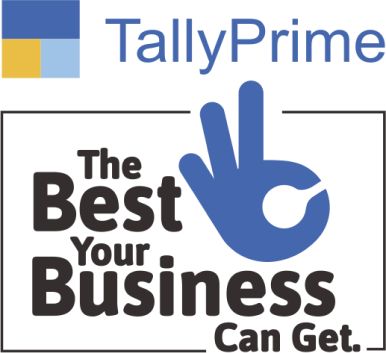# Book Value: Definition, Calculation and Example

## Book Value: Definition

Book value or carrying value could be defined as the net worth of an asset that is recorded on the balance sheet and it is simply calculated by subtracting any accumulated depreciation from an asset’s purchase price or the historical cost.

## Book value calculation

Usually, an assets book value is the current value of the asset with respect to the asset’s useful life. The whole calculation of book value adjusts the historical cost of an asset by the accumulated depreciation and we can arrive at the formula below:

## Components derived from Book Value Calculation

### Historical Cost

Historical cost is a replacement for the term cost. Historical cost generally means the original cost at the time of a transaction.

It distinguishes an asset's cost from its replacement cost, current cost, or inflation-adjusted cost.

The principle followed in ascertaining the historical cost of the asset requires that an asset should be reported at its cash or cash equivalent amount at the time of the transaction and should incorporate all the costs that are necessary to get the asset in place and ready for use.

### Example of Historical Cost

XYZ co Ltd a company purchased a property in 1989 for 1,00,000. It continues to own the property and has not made any improvements.

Therefore, the company should report the property purchased in balance sheet at its historical cost of 1,00,000 even though its current cost, replacement cost, inflation-adjusted cost, appraised value, and assessed value could be different.

### Accumulated Deprection

The sum total of the depreciation for a fixed asset is referred to as accumulated depreciation. It is treated as an expense. These are asset accounts with a credit balance known as a contra asset account. It materializes on the balance sheet as a deduction from the gross amount of fixed assets reported.

Let’s understand with an example.

ABC co., Ltd bought Machinery for 100,000 three years ago. The Machinery depreciates by 10,000 a year.

Thus, the accumulated depreciation recorded for the Machinery works out to be as follows:

 Time Year 1 Year2 Year 3 Accumulated Depreciation Depreciation Value 10,000 10,000 10,000 30,000

Note: Straight-line method of depreciation is followed here.

The netbook value or book value of machinery in books of ABC co., ltd by applying the book value calculation formulae is shown below:

 Metrics Amount Historical Cost 1,00,000 Less: Accumulated Depreciation 30,000 Book Value of Asset or Net Book Value 70,000

There is another method by which accumulated depreciation could be ascertained and it’s called Declining method or Written down value (WDV ) method of depreciation. This method is used to recognize the majority of an asset’s depreciation early in its lifespan.

Under Written down value method, depreciation amount changes from year to year and so it is more complicated to calculate than the straight-line method.

In this method depreciation is charged on the book value of asset. Let’s understand this with an example.

ABC and Bros purchased a Machinery for Rs. 1,00,000 and recorded its value in the balance sheet at the end of the third year to be Rs. 72,900. The rate of depreciation is 10%.

The working could be ascertained as follows:

 Time Workings Accumulated Depreciation at the end of 3rd year Year 1 Rs.1,00,000 * 10%. = Rs. 10,000 Rs. 10,000 Written down value ( WDV )  at the end of Year 1 B.V (Book Value)    1,00,000 Less: Acc.Dep.    10,000 N.B.V.    90,000 Year 2 Rs.90,000 * 10%. = Rs. 9,000 10,000 (+) 9000 = 19,000. Written down value ( WDV )  at the end of Year 2 B.V (Book Value)    90,000 Less: Acc.Dep.    9,000 N.B.V.    81,000 Year 3 Rs.81,000 * 10%. = Rs. 9,000 10,000 (+) 9000 (+) 8,100 = 27,100 Written down value ( WDV )  at the end of Year 3 B.V (Book Value)    81,000 Less: Acc.Dep.    8,100 N.B.V.    72,900

## Why calculating Book value is so important

Investors generally take an objective route of where a company stands in comparison to the market. Looking at other measures of valuation, the investors can get distracted and they may not be in a position to ascertain the true value of the company for them to decide whether to hold their stakes invested in the company or to liquidate it.

## Limitations of Book Value

One of the limitations of book value does not take into account Intellectual or Intangible Assets that a company posses.

Unlike physical assets such as machinery and buildings or financial assets such as government securities, intangible asset lacks physical substance. And it is also usually very hard to evaluate them.

Language

#### Latest BlogsBoost growth with affordable accounting. Take control of your finances today!